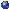The Bertini irreducibility theorem states that if X in P^N is a geometrically irreducible projective variety, then the general hyperplane section of X is geometrically irreducible. Benoist proved a stronger form of this theorem: if X has dimension n, the locus of hyperplanes H such that the hyperplane section X_H is geometrically reducible has codimension at least n-1 in the space of all hyperplanes. Poonen-Slavov gave an alternate proof of this result using point counts over finite fields. Their method gives a heuristic justification for a conjecture that the Gysin maps on cohomology induced by the inclusions of X_H in X are isomorphisms except in high codimension or low degree. In this talk I will explain the method of Poonen-Slavov and the conjecture for which it gives evidence. I will then sketch my proof of this conjecture in characteristic 0.